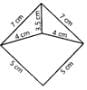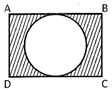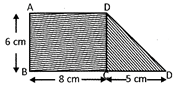Test : Area - 2

# Test : Area - 2

Test Description

## 10 Questions MCQ Test Mathematics for Class 5: NCERT | Test : Area - 2

Test : Area - 2 for Class 5 2022 is part of Mathematics for Class 5: NCERT preparation. The Test : Area - 2 questions and answers have been prepared according to the Class 5 exam syllabus.The Test : Area - 2 MCQs are made for Class 5 2022 Exam. Find important definitions, questions, notes, meanings, examples, exercises, MCQs and online tests for Test : Area - 2 below.
Solutions of Test : Area - 2 questions in English are available as part of our Mathematics for Class 5: NCERT for Class 5 & Test : Area - 2 solutions in Hindi for Mathematics for Class 5: NCERT course. Download more important topics, notes, lectures and mock test series for Class 5 Exam by signing up for free. Attempt Test : Area - 2 | 10 questions in 10 minutes | Mock test for Class 5 preparation | Free important questions MCQ to study Mathematics for Class 5: NCERT for Class 5 Exam | Download free PDF with solutions
 1 Crore+ students have signed up on EduRev. Have you?
Test : Area - 2 - Question 1

### Perimeter of an isosceles triangle is 32 cm. If length of the unequal side is 13 cm, find the length of one of the equal sides of the triangle.

Detailed Solution for Test : Area - 2 - Question 1

Perimeter of an isoclees triangle=32.
In an isoclees triangle two sides are equal.
the another side was different
here in the question they gave the unequal side length,which is13cm=y(say).
therefore 2x+y=32
2x+13=32
2x=32-13
2x=19
X=19/2
X=9.5

Test : Area - 2 - Question 2

### Find the perimeter of the circle whose diameter is 1.4 cm.

Test : Area - 2 - Question 3

### Find the area of the triangle whose base is 15 cm and height is 20 cm.

Test : Area - 2 - Question 4

Find the perimeter of the following figure.Test : Area - 2 - Question 5

Perimeter of a square is 60 cm. Find the area of the square.

Test : Area - 2 - Question 6

Look at the following figure:ABCD is a rectangle whose length and breadth are 16 cm and 14 cm respectively. Inside the rectangle ABCD, there is a circle. Find the area of the shaded region.

Test : Area - 2 - Question 7

Perimeter of a circle is 1.1 cm. Find the area of the circle.

Test : Area - 2 - Question 8

Base of a triangle is 2 cm more than the height. If height of the triangle is 12 cm, find the area of the triangle.

Test : Area - 2 - Question 9

Perimeter of a square is equal to the perimeter of the rectangle. If length and breadth of the rectangle is 24 cm and 20 respectively. Find the area of the square.

Test : Area - 2 - Question 10

Look at the following figure:ABCD is a rectangle. Find the area of the figure.

## Mathematics for Class 5: NCERT

33 videos|59 docs|39 tests
 Use Code STAYHOME200 and get INR 200 additional OFF Use Coupon Code
Information about Test : Area - 2 Page
In this test you can find the Exam questions for Test : Area - 2 solved & explained in the simplest way possible. Besides giving Questions and answers for Test : Area - 2, EduRev gives you an ample number of Online tests for practice

## Mathematics for Class 5: NCERT

33 videos|59 docs|39 tests